# Base

(diff) ← Older revision | Latest revision (diff) | Newer revision → (diff)

of a topological space, base of a topology, basis of a topology, open base

A family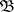of open subsets ofsuch that each open subsetis a union of subcollections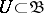. The concept of a base is a fundamental concept in topology: in many problems concerned with open sets of some space it is sufficient to restrict the considerations to its base. A space can have many bases, the largest one of which is the family of all open sets. The minimum of the cardinalities of all bases is called the weight of the topological space. In a space of weightthere exists an everywhere-dense set of cardinality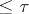. Spaces with a countable base are also referred to as spaces satisfying the second axiom of countability. The dual concept of a closed base, formed by the complements of the elements of a base, is not used to any significant extent.

A local base of a spaceat a point(a base of the point) is a family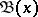of open sets ofwith the following property: For any neighbourhoodofit is possible to find an elementsuch that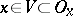. Spaces with a countable local base at every point are also referred to as spaces satisfying the first axiom of countability. A familyof open sets inis a base if and only if it is a local base of each one of its points.

Let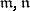be cardinal numbers. A baseof the spaceis called an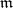-point base if each pointbelongs to at mostelements of the family; in particular, if, the base is called disjoint; ifis finite, it is called bounded point finite; and if, it is called point countable.

A baseof the spaceis called-local if each pointhas a neighbourhood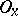intersecting with at mostelements of the family; in particular, if, the base is referred to as discrete; ifis finite, it is called bounded locally finite; and if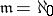, it is called locally countable. A baseis called an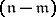-point base (or an-local base) if it is a union of a set of cardinalityof-point (-local) bases; examples are, for,-disjoint,-point finite,-discrete and-locally finite bases.

These concepts are used mainly in the criteria of metrizable spaces. Thus, a regular space with a countable base, or satisfying the first axiom of countability and with a point countable base, is metrizable; a regular space with a-discrete or-locally finite base is metrizable (the converse proposition is true in the former case only).

A baseof the spaceis called uniform (-uniform) if for each point(each compact subset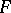) and for each one of the neighbourhoods(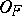) only a finite number of elements of the base contain(intersect with) and at the same time intersect with the complement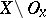(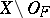). A spaceis metrizable if and only if it is paracompact with a uniform base (a Kolmogorov or-space with a-uniform base).

A baseof the spaceis called regular if for each pointand an arbitrary neighbourhoodof it there exists a neighbourhood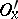such that the set of all the elements of the base which intersect both withandis finite. An accessible or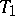-space is metrizable if and only if it has a regular base.

A generalization of the concept of a base is the so-called-base (lattice base), which is a familyof open sets in the spacesuch that each non-empty open set incontains a non-empty set from, i.e.is dense inaccording to Hausdorff. All bases are-bases, but the converse is not true; thus, the set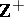in the Stone–Čech compactification of the set of natural numbers informs only a-base.

How to Cite This Entry:
Base. Encyclopedia of Mathematics. URL: http://encyclopediaofmath.org/index.php?title=Base&oldid=12315
This article was adapted from an original article by A.A. Mal'tsev (originator), which appeared in Encyclopedia of Mathematics - ISBN 1402006098. See original article